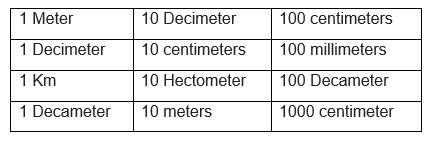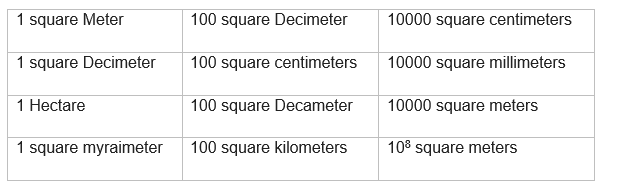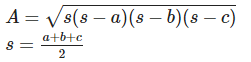# Heron's Formula Class 9 Notes

Welcome to Class 9 Maths chapter 12 Heron's Formula notes.The topics in this page are Perimeter,Area of triangle using Heron's Formula, Perimeter and Area of Different Figure.This is according to CBSE and the NCERT textbook. If you like the study material, feel free to share the link as much as possible.

Table of Content

## Mensuration

• It is branch of mathematics which is concerned about the measurement of length ,area and Volume of plane and Solid figure

## Perimeter

• The perimeter of plane figure is defined as the length of the boundary
• It units is same as that of length i.e. m ,cm,kmNote:
You have read from Left to right to get the conversion formula
1 m= 10 dm = 100 cm
1 dm= 10 cm = 100 mm
1 km= 10 hm = 100 decameters
1 decameter= 10 m = 1000 cm

## Area

• The area of the plane figure is the surface enclosed by its boundary
• It unit is square of length unit. i.e. m2 , km2Note:
You have read from Left to right to get the conversion formula
$1 m^2 = 100 dm^2 = 10^4 cm^2$
$1 dm^2 = 100 cm^2 = 10^4 mm^2$
$1 \ hectare = 100 \ square \ Decameter = 10^2 m^2$
$1 \ square \ myraimeter=100 km^2 = 10^8 m^2$

## Perimeter and Area of Different Figure

 N Shape Perimeter/height Area 1 Right angle triangle Base =b, Height =h Hypotenuse=d $P=b+h+d$ Height =h $A=\frac {1}{2}BH$ 2 Isosceles right angled triangle Equal side =a $P= a(2+ \sqrt 2)$ $A=\frac {1}{2} a^2$ 3 Isosceles triangle Equal side =a and base as b $P= 2a +b$ $A=\frac {1}{2} a \sqrt {a^2 - \frac {b^2}{4}}$ 4 Any Triangle Any triangle of sides a,b ,c $P=a+b+c$ $A=\sqrt {s(s-a)(s-b)(s-c)}$ $s=\frac {a+b+c}{2}$ This is called Heron's formula (sometimes called Hero's formula) is named after Hero of Alexandria 5 Square Side =a P=4a $A=a^2$ 6 Rectangle of Length and breath L and B respectively $P=2L +2B$ $A=L \times B$ 7 Parallelograms Two sides are given as a and b $P=2a+2b$ $A= Base \times height$ When the diagonal is also given ,say d Then $A= \sqrt {s(s-a)(s-b)(s-d)}$ $s=\frac {a+b+d}{2}$ 8 Rhombus Diagonal d1 and d2 are given $p=2 \sqrt {d_1^2+d_2^2}$ Each side=$\frac {1}{2} \sqrt {d_1^2+d_2^2}$ $A=\frac {1}{2} d_1 d_2$ 9 Quadrilateral a. All the sides are given a,b,c ,d b. Both the diagonal are perpendicular to each other c. When a diagonal and perpendicular to diagonal are given a. $P=a+b+c+d$ a. $A=\sqrt {s(s-a)(s-b)(s-c)(s-d)}$ $s=\frac {(a+b+c+d)}{2}$ b. $A=\frac {1}{2} d_1 d_2$ where d1 and d2 are the diagonal c. $A=\frac {1}{2} d(h_1+h_2)$ where d is diagonal and h1 and h2 are perpendicular to that

## How to solve the Area and Perimeter problems

1. We must remember the formula for all the common figures as given above the table
2. Find out what all is given in the problem
3. Convert all the given quantities in the same unit
4. Sometimes Perimeter is given and some side is unknown,So you can calculate the sides using the Perimeter
5. If it is a complex figure ,break down into common know figures like square,rectangle,triangle
6. Sometimes we can find another side using Pythagoras theorem in the complex figure
7. If common figure, apply the formula given above and calculate the area.
8. If complex figure, calculate the area for each common figure in it and sum all the area at the end to calculate the total area of the figure

## Solved Examples

Question 1.
A right angle triangle has base 20 cm and height as 10 cm, What is the area of the triangle?
Solution
Given values B=20 cm
H=10 cm
Both are in same units
$A=\frac {1}{2}BH=100 \ cm^2$

Question 2.
Sides of triangles are in the ratio 12:17:25. The perimeter of the triangle is 540 cm. Find out the area of the triangle?
Solution
Let the common ration between the sides be y,then sides are 12y,17y,25 y
Now we know the perimeter of the triangle is given by
P=a+b+c
540=12y+17y+25y
or y=10 cm
Now Area of triangle is given as $A=\sqrt {s(s-a)(s-b)(s-c)}$
Where s=(a+b+c)/2
Here s=270 cm
a=120 cm
b=170cm
c=250cm
Substituting all these values in the area equation,we get
A=9000 cm2

Question 3.
A equilateral triangle is having side 2 cm. What is the area of the triangle?
Solution
We know that Are of equilateral triangle is given by
$A= \frac {a^2 \sqrt 3}{4}$
Substituting the values given above
$A=\sqrt 3 \ cm^2$

## We can summarize various method to calculate the Area of the Triangle

 If you know the altitude and Base $A =\frac {1}{2}BH$ If you all the three sides $A=\sqrt {s(s-a)(s-b)(s-c)}$ $s=\frac {a+b+c}{2}$ If it is isosceles right angle triangle with equal side a $A=\frac {1}{2}a^2$ If it is isosceles triangle with equal side a and other side as $A=\frac {1}{2} a \sqrt {a^2 - \frac {b^2}{4}}$ If it is equilateral triangle with equal side a $A=\frac {a^2 \sqrt 3}{4}$ If it is right angle triangle with Base B and Height H $A =\frac {1}{2}BH$

## Summary

Here is Heron's Formula Class 9 Notes summary
• Area of a triangle with its sides as a, b and c is calculated by using Heron’s formula,• Area of a quadrilateral whose sides and one diagonal are given, can be calculated by dividing the quadrilateral into two triangles and using the Heron’s formula